Technical Test SSC JE: Electrical Engineering (EE)- 3

# Technical Test SSC JE: Electrical Engineering (EE)- 3

Test Description

## 100 Questions MCQ Test Mock Test Series for SSC JE Electrical Engineering | Technical Test SSC JE: Electrical Engineering (EE)- 3

Technical Test SSC JE: Electrical Engineering (EE)- 3 for Electrical Engineering (EE) 2022 is part of Mock Test Series for SSC JE Electrical Engineering preparation. The Technical Test SSC JE: Electrical Engineering (EE)- 3 questions and answers have been prepared according to the Electrical Engineering (EE) exam syllabus.The Technical Test SSC JE: Electrical Engineering (EE)- 3 MCQs are made for Electrical Engineering (EE) 2022 Exam. Find important definitions, questions, notes, meanings, examples, exercises, MCQs and online tests for Technical Test SSC JE: Electrical Engineering (EE)- 3 below.
Solutions of Technical Test SSC JE: Electrical Engineering (EE)- 3 questions in English are available as part of our Mock Test Series for SSC JE Electrical Engineering for Electrical Engineering (EE) & Technical Test SSC JE: Electrical Engineering (EE)- 3 solutions in Hindi for Mock Test Series for SSC JE Electrical Engineering course. Download more important topics, notes, lectures and mock test series for Electrical Engineering (EE) Exam by signing up for free. Attempt Technical Test SSC JE: Electrical Engineering (EE)- 3 | 100 questions in 100 minutes | Mock test for Electrical Engineering (EE) preparation | Free important questions MCQ to study Mock Test Series for SSC JE Electrical Engineering for Electrical Engineering (EE) Exam | Download free PDF with solutions
 1 Crore+ students have signed up on EduRev. Have you?
Technical Test SSC JE: Electrical Engineering (EE)- 3 - Question 1

### A cube of material of side 1 cm has a resistance of 0.002W between its opposite faces. If the same volume of the material has a length of 8 cm and a uniform cross-section the resistanceof this length will be

Detailed Solution for Technical Test SSC JE: Electrical Engineering (EE)- 3 - Question 1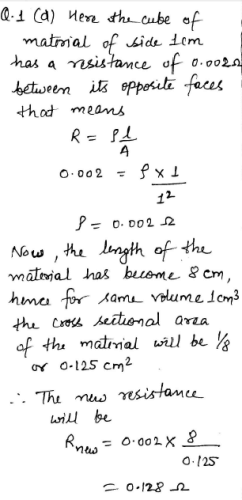Technical Test SSC JE: Electrical Engineering (EE)- 3 - Question 2

### The incandescent bulb rated respectively as P1 and P2 for operation at a specified main voltage are connected in series across the mains as shown in the figure. Then the total power supplied by the mains to the two bulbs.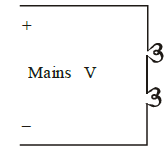Detailed Solution for Technical Test SSC JE: Electrical Engineering (EE)- 3 - Question 2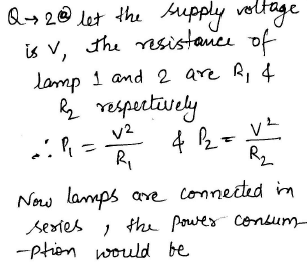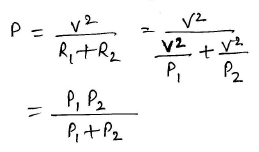Technical Test SSC JE: Electrical Engineering (EE)- 3 - Question 3

### A metal filament lamp X rated 40 W, 100 V is connected in series with another lamp Y of the same type rated 100W, 200V. A voltage of 200V is applied across the combination then

Detailed Solution for Technical Test SSC JE: Electrical Engineering (EE)- 3 - Question 3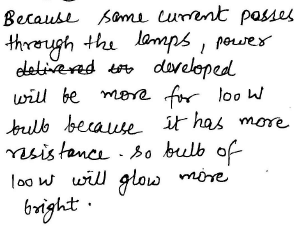Technical Test SSC JE: Electrical Engineering (EE)- 3 - Question 4

A 100W, 200V, filament lamp has operating temperature of 2000ºC. The filament materialhas resistance temperature coefficient of 0.005 at OºC per ºC. The current taken by the lamp at the instant of switching with 200V supply with filament temperature of 20ºC will be

Detailed Solution for Technical Test SSC JE: Electrical Engineering (EE)- 3 - Question 4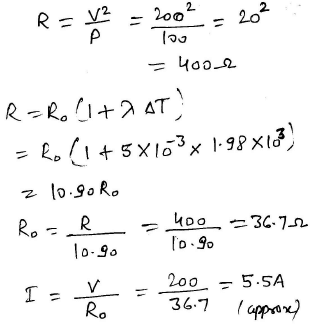Technical Test SSC JE: Electrical Engineering (EE)- 3 - Question 5

The equivalent resistance at 'the points X1 and X2 in the circuits shown below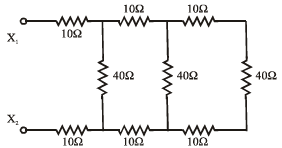Technical Test SSC JE: Electrical Engineering (EE)- 3 - Question 6

In the circuit shown in the given figure. The current through the inductor L is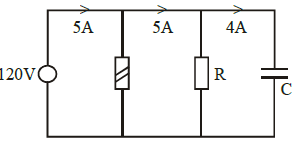Detailed Solution for Technical Test SSC JE: Electrical Engineering (EE)- 3 - Question 6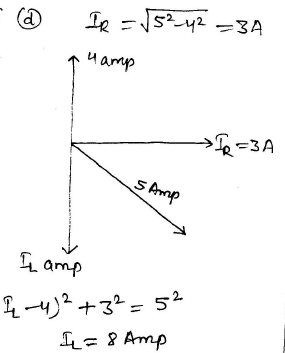Technical Test SSC JE: Electrical Engineering (EE)- 3 - Question 7

A series RLC circuit is switched ON to a step voltage 'V' at t=0. What are initial and finalvalues of the current in the circuit respectively?

Detailed Solution for Technical Test SSC JE: Electrical Engineering (EE)- 3 - Question 7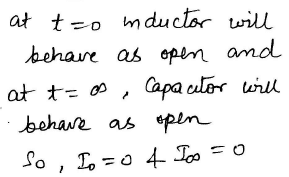Technical Test SSC JE: Electrical Engineering (EE)- 3 - Question 8

(1) Under steady state condition, a pure inductor act as a short circuit for direct current

(2) The potential drop across an inductance is proportional to the rate of change of current

Technical Test SSC JE: Electrical Engineering (EE)- 3 - Question 9

According to maximum power transfer theorem, when is the maximum power absorbed by onenetwork from another network ?

Detailed Solution for Technical Test SSC JE: Electrical Engineering (EE)- 3 - Question 9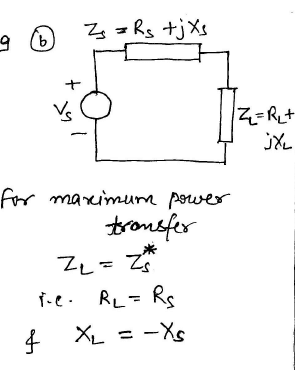Technical Test SSC JE: Electrical Engineering (EE)- 3 - Question 10

At resonant frequency a R-L-C series circuit draws maximum current due to the reason that

Technical Test SSC JE: Electrical Engineering (EE)- 3 - Question 11

If for sinusoids of same frequency but ofdifferent amplitudes and phase difference areadded the resultant is a

Technical Test SSC JE: Electrical Engineering (EE)- 3 - Question 12

A pure capacitance connected across 50Hz, 230V supply consumes 0.04W. This consumption is attributed to

Technical Test SSC JE: Electrical Engineering (EE)- 3 - Question 13

A 2-terminal network consist of one of the RLC elements. The element is connected to an ac supply. The current through the element is IA when an inductor is inserted in series between the source and element 2IA the current through the element becomes. What is this element ?

Technical Test SSC JE: Electrical Engineering (EE)- 3 - Question 14

With the increase in applied frequency, thedielectric loss in a material will

Technical Test SSC JE: Electrical Engineering (EE)- 3 - Question 15

A 100W, 100V bulb is to be supplied from 220V, 50Hz supply. Which of the following arrangement is preferable ?

Technical Test SSC JE: Electrical Engineering (EE)- 3 - Question 16

A small capacitance is added to a highly inductive circuit

Technical Test SSC JE: Electrical Engineering (EE)- 3 - Question 17

In an RLC circuit

Technical Test SSC JE: Electrical Engineering (EE)- 3 - Question 18

Capacitive susceptance is a measure of

Technical Test SSC JE: Electrical Engineering (EE)- 3 - Question 19

Q-factor of a series R-L-C circuit possessing resonant frequency of 10Hz and bandwidth of 5Hz is

Technical Test SSC JE: Electrical Engineering (EE)- 3 - Question 20

A parallel circuit is said to be in resonance when the admittance is purely

Technical Test SSC JE: Electrical Engineering (EE)- 3 - Question 21

A conductor of length 100 cm moves at right angle to a uniform field flux density of 1.5 W6/m2 with a velocity of 50 m/s. The emf induced in the conductor will be

Detailed Solution for Technical Test SSC JE: Electrical Engineering (EE)- 3 - Question 21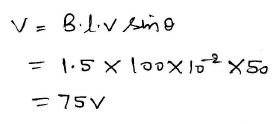Technical Test SSC JE: Electrical Engineering (EE)- 3 - Question 22

Magnetic materials which may be readily magnetized in either direction are

Technical Test SSC JE: Electrical Engineering (EE)- 3 - Question 23

The relative permeability is less than unity in case of

Technical Test SSC JE: Electrical Engineering (EE)- 3 - Question 24

A 1000 turn solenoid develops an average induced voltage of 120 volts. Over what timeinterval must a flux change of 60 milli-webers occurs to produce such a voltage ?

Detailed Solution for Technical Test SSC JE: Electrical Engineering (EE)- 3 - Question 24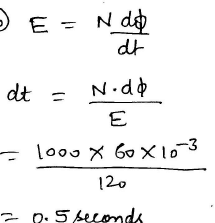Technical Test SSC JE: Electrical Engineering (EE)- 3 - Question 25

A long solenoid with 2000 turns is 0.8 m long. What is the magnetising force in the centre of this solenoid, if the exciting current is 2A

Detailed Solution for Technical Test SSC JE: Electrical Engineering (EE)- 3 - Question 25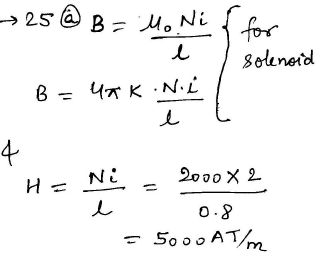Technical Test SSC JE: Electrical Engineering (EE)- 3 - Question 26

For a D.C. machine laboratory following type of DC supply will be used

Technical Test SSC JE: Electrical Engineering (EE)- 3 - Question 27

Lamination of core are generally made of

Technical Test SSC JE: Electrical Engineering (EE)- 3 - Question 28

The speed control of dc shunt motor in both directions can be obtained by

Technical Test SSC JE: Electrical Engineering (EE)- 3 - Question 29

A synchronous motor operates at rated voltageand rated frequency and has a load torque angle of 30º. If both the terminal voltage & frequency are reduced by 10%, then

Detailed Solution for Technical Test SSC JE: Electrical Engineering (EE)- 3 - Question 29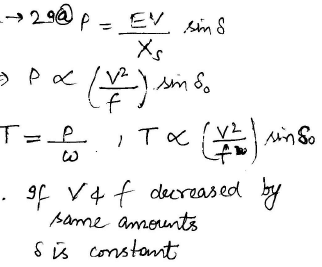Technical Test SSC JE: Electrical Engineering (EE)- 3 - Question 30

The nature of armature mmf for a zero power factor lagging load in a 3-f alternator is

Detailed Solution for Technical Test SSC JE: Electrical Engineering (EE)- 3 - Question 30

Explanation : The effect of armature reaction mmf is purely demagnetizing in zero pf lagging load condition. This can be explained in 2 methods :

In Zero power factor lagging load, the load current is lagging behind the excitation voltage by 90 degree.

Due to zero power factor lagging load, the current in phase “a” of armature winding will become maximum when the field poles have advanced by 90 degree in assumed counter-clockwise direction.

Technical Test SSC JE: Electrical Engineering (EE)- 3 - Question 31

The frequency of rotor emf of an 8-pole induction motor is 24HZ. If the supply frequency is 50Hz, then the motor speed is

Detailed Solution for Technical Test SSC JE: Electrical Engineering (EE)- 3 - Question 31

Explanation :

Number of poles (P) = 8

Frequency (f) = 50

N₁ = 120x50 = 750 rpm

Frequency of rotor emf = sf = 2 Hz

Supply frequency = f = 50 Hz

⇒ s = 0.04

Rotor speed = Ns (1 s) = 750 (1-0.04) = 720 rpm

Technical Test SSC JE: Electrical Engineering (EE)- 3 - Question 32

For a given applied voltage and current, the speed of a universal motor will be

Detailed Solution for Technical Test SSC JE: Electrical Engineering (EE)- 3 - Question 32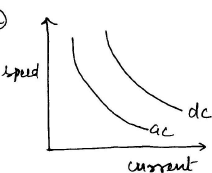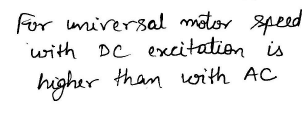Technical Test SSC JE: Electrical Engineering (EE)- 3 - Question 33

Which of the following is the main advantage of an auto-transformer over a two winding transformer ?

Technical Test SSC JE: Electrical Engineering (EE)- 3 - Question 34

When a given transformer is run at a its rated voltage but reduced frequency its

Technical Test SSC JE: Electrical Engineering (EE)- 3 - Question 35

A 2000/200V, 50 Hz single phase transformer has an exciting current of 0.5A and a core loss of 600W. When H.V. side is energized by the rated voltage and frequency, the magnetisingcurrent is

Detailed Solution for Technical Test SSC JE: Electrical Engineering (EE)- 3 - Question 35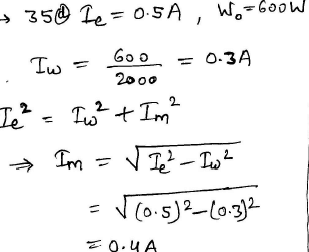Technical Test SSC JE: Electrical Engineering (EE)- 3 - Question 36

In case of dc motor, maximum mechanical power is developed, when back emf equals

Technical Test SSC JE: Electrical Engineering (EE)- 3 - Question 37

If Pc & Psc represent core & full-load ohmic losses respectively, the maximum KVAdelivered to load corresponding to maximum efficiency is equal to rated KVA multiplied by

Technical Test SSC JE: Electrical Engineering (EE)- 3 - Question 38

Under no load conditions, which of the following loss is negligible ?

Technical Test SSC JE: Electrical Engineering (EE)- 3 - Question 39

Open circuit test of a transformer gives

Technical Test SSC JE: Electrical Engineering (EE)- 3 - Question 40

Detailed Solution for Technical Test SSC JE: Electrical Engineering (EE)- 3 - Question 40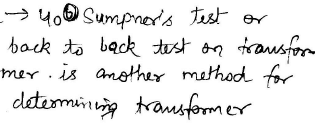Technical Test SSC JE: Electrical Engineering (EE)- 3 - Question 41

If flux density in the core of a transformer is increased

Technical Test SSC JE: Electrical Engineering (EE)- 3 - Question 42

The driving power from the prime mover driving the alternator is lost but the alternator remainsconnected to the supply network and the field supply also remains on. The alternator will

Technical Test SSC JE: Electrical Engineering (EE)- 3 - Question 43

Two alternators are running in parallel. If the driving force of both the alternators is changed this will result in change is

Technical Test SSC JE: Electrical Engineering (EE)- 3 - Question 44

The armature reaction of an alternator influences

Technical Test SSC JE: Electrical Engineering (EE)- 3 - Question 45

In an alternator if the armature reaction produces magnetisation of the main field the power factor should be

Detailed Solution for Technical Test SSC JE: Electrical Engineering (EE)- 3 - Question 45

Explanation : Zero Power factor means that either the circuit is purely resistive or purely capacitive.

If the load is purely capacitive or at the leading power factor, the effect of armature reaction is of magnetizing type.

Note:- In the magnetizing effect, armature flux assists main field flux hence greater EMF is induced in the armature. Hence there is an increase in the terminal voltage for leading power factor loads

Technical Test SSC JE: Electrical Engineering (EE)- 3 - Question 46

As the load on an induction motor increases

Technical Test SSC JE: Electrical Engineering (EE)- 3 - Question 47

A 3-f 420V, 60Hz induction motor is to be run on 50 Hz supply. Which of the following will be the satisfactory supply voltage for the machine ?

Detailed Solution for Technical Test SSC JE: Electrical Engineering (EE)- 3 - Question 47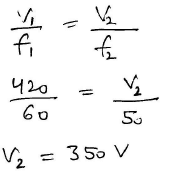Technical Test SSC JE: Electrical Engineering (EE)- 3 - Question 48

The torque of a reluctance motor can be effectively increased by

Technical Test SSC JE: Electrical Engineering (EE)- 3 - Question 49

A 2-phase servomotor in control system uses

Technical Test SSC JE: Electrical Engineering (EE)- 3 - Question 50

In dc machines, the field flux axis and the armature mmf axis are respectively along

Technical Test SSC JE: Electrical Engineering (EE)- 3 - Question 51

A power station has a maximum demand of 2500 kw and number of kwh generated per year is 45×105. The load factor is

Detailed Solution for Technical Test SSC JE: Electrical Engineering (EE)- 3 - Question 51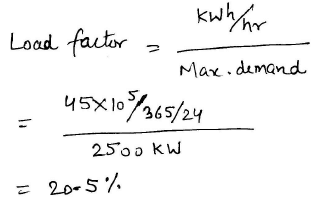Technical Test SSC JE: Electrical Engineering (EE)- 3 - Question 52

A long overhead losses power transmission line is terminated with its characteristic impedance while the line is in operation

Technical Test SSC JE: Electrical Engineering (EE)- 3 - Question 53

In case of single line to ground fault

Technical Test SSC JE: Electrical Engineering (EE)- 3 - Question 54

Which of the following circuit breakers has the lowest voltage range ?

Detailed Solution for Technical Test SSC JE: Electrical Engineering (EE)- 3 - Question 54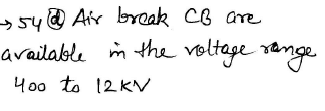Technical Test SSC JE: Electrical Engineering (EE)- 3 - Question 55

Which of the following will be provided to reduce the harmonics on the a.c. side of an HVDC transmission line ?

Technical Test SSC JE: Electrical Engineering (EE)- 3 - Question 56

Which distribution system is more reliable ?

Technical Test SSC JE: Electrical Engineering (EE)- 3 - Question 57

Which of the following circuit breakers does not use pneumatic operating mechanism

Technical Test SSC JE: Electrical Engineering (EE)- 3 - Question 58

A relay used for protection of motors against overload is

Technical Test SSC JE: Electrical Engineering (EE)- 3 - Question 59

Switching over-voltages are more hazardous than lightening surges in case of

Technical Test SSC JE: Electrical Engineering (EE)- 3 - Question 60

Series reactors are installed at strategic locations of power systems to

Technical Test SSC JE: Electrical Engineering (EE)- 3 - Question 61

Single core cables are usually not provided with

Technical Test SSC JE: Electrical Engineering (EE)- 3 - Question 62

Copper as conductors for cables is used as

Technical Test SSC JE: Electrical Engineering (EE)- 3 - Question 63

If two synchronous generators are connected loss of synchronism will result in

Detailed Solution for Technical Test SSC JE: Electrical Engineering (EE)- 3 - Question 63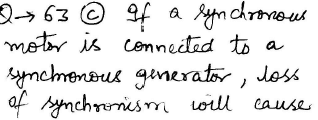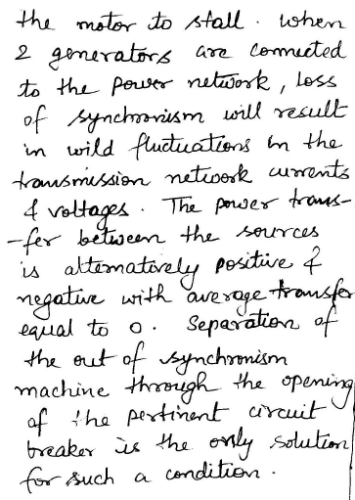Technical Test SSC JE: Electrical Engineering (EE)- 3 - Question 64

The maximum demand of a power station is

Technical Test SSC JE: Electrical Engineering (EE)- 3 - Question 65

Static capacitor are rated in terms of

Technical Test SSC JE: Electrical Engineering (EE)- 3 - Question 66

High voltage transmission lines are transposed because then

Technical Test SSC JE: Electrical Engineering (EE)- 3 - Question 67

The power factor of an open ended cable can be improved by

Technical Test SSC JE: Electrical Engineering (EE)- 3 - Question 68

The maximum short circuit current occurs in case of

Technical Test SSC JE: Electrical Engineering (EE)- 3 - Question 69

A fault occurring at the terminal of an unloaded synchronous generator operating at its rated voltage has resulted in the following values of currents and voltages

Iao = j2.37Pu Ia1 = j3.05 Pu Ia2 = j0.68 Pu

Technical Test SSC JE: Electrical Engineering (EE)- 3 - Question 70

In an overcurrent protection the retting of the earth fault relay is

Technical Test SSC JE: Electrical Engineering (EE)- 3 - Question 71

Time response of an indicating instrument is decided by which of the following systems ?

Technical Test SSC JE: Electrical Engineering (EE)- 3 - Question 72

A 50 mA basic d'Arsonval movement with an internal resistance of 500W is to be used as a voltmeter. The value of the multiplier resistance required to measure a full scale voltage range of 0-5 volts is

Detailed Solution for Technical Test SSC JE: Electrical Engineering (EE)- 3 - Question 72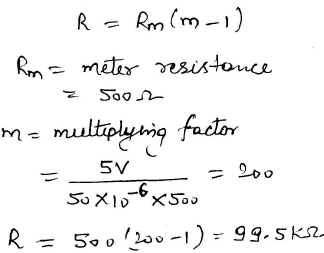Technical Test SSC JE: Electrical Engineering (EE)- 3 - Question 73

In measuring resistance by voltmeter ammeter method, the voltmeter can be connected either across supply or across the resistance. If the resistance is low, the voltmeter should be connected

Technical Test SSC JE: Electrical Engineering (EE)- 3 - Question 74

A dual beam CRO

Technical Test SSC JE: Electrical Engineering (EE)- 3 - Question 75

A Wheatstone bridge has got three resistances taken in clockwise direction as 120W, 150W & 150W. The value of fourth resistance for null balance would be

Detailed Solution for Technical Test SSC JE: Electrical Engineering (EE)- 3 - Question 75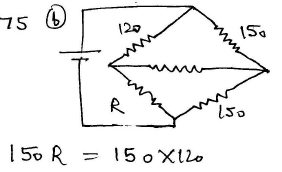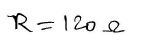Technical Test SSC JE: Electrical Engineering (EE)- 3 - Question 76

Inductance is measured by which one of the following ?

Detailed Solution for Technical Test SSC JE: Electrical Engineering (EE)- 3 - Question 76

Maxwell inductance capacitance bridge is used for the measurement of inductance by making comparison with a standard capacitance value. Voltmeter is used to measure voltage, while an ammeter is used to measure current.

Technical Test SSC JE: Electrical Engineering (EE)- 3 - Question 77

Electronic voltmeters which use rectifiers employ negative feedback. This is done

Technical Test SSC JE: Electrical Engineering (EE)- 3 - Question 78

Guard circuits are used in insulation resistance measurements to

Technical Test SSC JE: Electrical Engineering (EE)- 3 - Question 79

Which of the following instrument does not use the effect of current for measurement purposes?

Technical Test SSC JE: Electrical Engineering (EE)- 3 - Question 80

One wattmeter method is used to measure power in three circuits when

Technical Test SSC JE: Electrical Engineering (EE)- 3 - Question 81

In two wattmeter method, if power factor is 0.5, then one of the wattmeters will read

Technical Test SSC JE: Electrical Engineering (EE)- 3 - Question 82

Which is the integrating instrument ?

Technical Test SSC JE: Electrical Engineering (EE)- 3 - Question 83

The ratio error of a current transformer is due to

Technical Test SSC JE: Electrical Engineering (EE)- 3 - Question 84

An electrodynamic wattmeter is not considered suitable for low power factor circuits due to

Technical Test SSC JE: Electrical Engineering (EE)- 3 - Question 85

A Q-meter works on the principle of

Technical Test SSC JE: Electrical Engineering (EE)- 3 - Question 86

Which instrument has the same calibration for ac & dc values ?

Technical Test SSC JE: Electrical Engineering (EE)- 3 - Question 87

Which voltmeter has least power consumption?

Technical Test SSC JE: Electrical Engineering (EE)- 3 - Question 88

A ballastic galvanometer is used for the measurement of

Technical Test SSC JE: Electrical Engineering (EE)- 3 - Question 89

The bridge used for the precise measurement of inductance over a wide range is

Technical Test SSC JE: Electrical Engineering (EE)- 3 - Question 90

When a transistor is connected in common emitter mode it will have

Technical Test SSC JE: Electrical Engineering (EE)- 3 - Question 91

A bridge used to measure the inductance haring large time constant is

Detailed Solution for Technical Test SSC JE: Electrical Engineering (EE)- 3 - Question 91

Hay’s Bridge is suited for the measurement of Inductance of a coil with a large time constant.

Technical Test SSC JE: Electrical Engineering (EE)- 3 - Question 92

The concentration of minority carries in an extrinsic semiconductor under equilibrium is

Technical Test SSC JE: Electrical Engineering (EE)- 3 - Question 93

For forward biased diode

Technical Test SSC JE: Electrical Engineering (EE)- 3 - Question 94

In a p-n junction diode under reverse bias, the magnitude of electric field is maximum at

Technical Test SSC JE: Electrical Engineering (EE)- 3 - Question 95

As compared to an LED, an LCD has the distinct advantage of

Technical Test SSC JE: Electrical Engineering (EE)- 3 - Question 96

CE configuration is the most preferred transistor configuration when used as a switch because it

Technical Test SSC JE: Electrical Engineering (EE)- 3 - Question 97

In a NPN transistor, when emitter junction is forward biased and collector junction is reverse biased, the transistor will operate in

Technical Test SSC JE: Electrical Engineering (EE)- 3 - Question 98

In normally biased NPN transistor the main current crossing the collector junction is

Technical Test SSC JE: Electrical Engineering (EE)- 3 - Question 99

Which junction transistor is preferred for high input and low output impedance ?

Technical Test SSC JE: Electrical Engineering (EE)- 3 - Question 100

A FET has

## Mock Test Series for SSC JE Electrical Engineering

2 videos|1 docs|55 tests
 Use Code STAYHOME200 and get INR 200 additional OFF Use Coupon Code
Information about Technical Test SSC JE: Electrical Engineering (EE)- 3 Page
In this test you can find the Exam questions for Technical Test SSC JE: Electrical Engineering (EE)- 3 solved & explained in the simplest way possible. Besides giving Questions and answers for Technical Test SSC JE: Electrical Engineering (EE)- 3, EduRev gives you an ample number of Online tests for practice

## Mock Test Series for SSC JE Electrical Engineering

2 videos|1 docs|55 tests